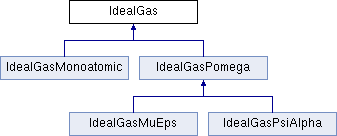JDFTx  1.7.0IdealGas Class Referenceabstract

Abstract base class for an IdealGas evaluator. More...

#include <IdealGas.h>

Inheritance diagram for IdealGas:## Public Member Functions

IdealGas (int nIndep, const FluidMixture *, const FluidComponent *)
Initialize and register to be used with excess functional fex in its fluidMixture.

virtual void initState (const ScalarField *Vex, ScalarField *indep, double scale, double Elo=-DBL_MAX, double Ehi=+DBL_MAX) const =0

virtual void getDensities (const ScalarField *indep, ScalarField *N, vector3<> &P0) const =0
Given the independent variables indep, compute the site densities N and G=0 component of polarization density P.

virtual double compute (const ScalarField *indep, const ScalarField *N, ScalarField *Phi_N, const double Nscale, double &Phi_Nscale) const =0

virtual void convertGradients (const ScalarField *indep, const ScalarField *N, const ScalarField *Phi_N, const vector3<> &Phi_P0, ScalarField *Phi_indep, const double Nscale) const =0

double get_Nbulk ()

void overrideBulk (double Nbulk, double mu)
Override the values for bulk density and chemical potential set by fluidMixture::initialize()

## Public Attributes

const int nIndep
Number of scalar fields used as independent variables.

const Moleculemolecule
Associated molecule geometry.

const GridInfogInfo
grid specifications

const double T
temperature

ScalarFieldArray V
external site potentials

## Protected Attributes

double Nbulk
equilibirum density of this molecule in the bulk mixture

double mu
chemical potential for this molecule

double corrPrefac
prefactor for dipolar rotational correlations

## Friends

class FluidMixture
FluidMixture::initialize() adjusts Nbulk and mu to get target pressure and mole fractions.

## Detailed Description

Abstract base class for an IdealGas evaluator.

## ◆ compute()

 virtual double IdealGas::compute ( const ScalarField * indep, const ScalarField * N, ScalarField * Phi_N, const double Nscale, double & Phi_Nscale ) const
pure virtual

Return the ideal gas free energy PhiNI = T Int N - T S + (V-mu).N (where S is implementation dependent) and accumulate the gradients w.r.t the site densities Nscale is the factor by which the site densities/moments were scaled after getDensities() in order to implement fixed N / charge neutrality. Accumulate explicit gradients of PhiNI w.r.t Nscale in Phi_Nscale; the implicit dependence through N is handled by FluidMixture.

Implemented in IdealGasMonoatomic, and IdealGasPomega.

 virtual void IdealGas::convertGradients ( const ScalarField * indep, const ScalarField * N, const ScalarField * Phi_N, const vector3<> & Phi_P0, ScalarField * Phi_indep, const double Nscale ) const
pure virtual

Compute Phi_indep, the total gradients w.r.t indep, given th egradients of the entire functional w.r.t site densities, Phi_N, and polarization density G=0 Phi_P0. Nscale will be the same as in compute()

Implemented in IdealGasMonoatomic, and IdealGasPomega.

## ◆ initState()

 virtual void IdealGas::initState ( const ScalarField * Vex, ScalarField * indep, double scale, double Elo = -DBL_MAX, double Ehi = +DBL_MAX ) const
pure virtual

Create an initial guess for the indep, in presence of V and the extra potential Vex The initial guess is typically taken to be scale times what would generate the equilibrium ideal gas density upto caps Elo and Ehi on the molecule energy configurations considered. This would also be a good place to logPrintf useful statistics about the potential for debugging

Implemented in IdealGasPsiAlpha, IdealGasMonoatomic, and IdealGasPomega.

The documentation for this class was generated from the following file:
• fluid/IdealGas.h